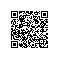# 快速排序中的分割算法的解析与应用1 //分割数组,将数组分成两部分. 一部分比pivot(枢轴元素)大,另一部分比pivot小
2     private static int parition(int[] arr, int left, int right){
3
4         int pivot = media3(arr, left, right);
5         int i = left;
6         int j = right - 1;//注意 ,在 media3()中 arr[right-1]就是 pivot
7
8         for(;;)
9         {
10             while(arr[++i] < pivot){}
11             while(arr[--j] > pivot){}
12             if(i < j)
13                 swap(arr, i, j);
14             else
15                 break;
16         }
17
18         swap(arr, i, right-1);//restore pivot， 将枢轴元素放置到合适位置:arr左边元素都比pivot小,右边都比pivot大
19         return i;// 返回 pivot的 索引
20     }①第4行，枢轴元素是通过“三数取中”法选择的。在“三数取中”时，还做了一些优化：将 枢轴元素 放到 数组末尾的倒数第二个位置处。具体参考 media3()1 //分割数组,将数组分成两部分. 一部分比pivot(枢轴元素)大,另一部分比pivot小
2     private static int parition(int[] arr, int left, int right){
3
4         int pivot = media3(arr, left, right);
5         int i = left;
6         int j = right - 1;//注意 ,在 media3()中 arr[right-1]就是 pivot
7
8         //应对特殊情况下的数组,比如数组长度 小于3
9         if(i >= j)
10             return i;
11
12         for(;;)
13         {
14             while(arr[++i] < pivot){}
15             while(arr[--j] > pivot){}
16             if(i < j)
17                 swap(arr, i, j);
18             else
19                 break;
20         }
21
22         swap(arr, i, right-1);//restore pivot 将枢轴元素放置到合适位置:arr左边元素都比pivot小,右边都比pivot大
23         return i;// 返回 pivot的 索引
24     }1     //三数取中,用在快排中随机选择枢轴元素时
2     private static int media3(int[] arr, int left, int right){
3         if(arr.length == 1)
4             return arr;
5
6         if(left == right)
7             return arr[left];
8
9         int center = (left + right) / 2;
10
11         //找出三个数中的最小值放到 arr[left]
12         if(arr[center] < arr[left])
13             swap(arr, left, center);
14         if(arr[right] < arr[left])
15             swap(arr, left, right);
16
17         //将 中间那个数放到 arr[media]
18         if(arr[center] > arr[right])
19             swap(arr, center, right);
20
21         swap(arr, center, right-1);//尽量将大的元素放到右边--将privot放到右边, 可简化 分割操作(partition).
22         return arr[right-1];//返回中间大小的那个数
23     }1 //找出 arr 中 第  n/2  大的那个元素
2     public static int media_number(int[] arr){
3         int left = 0;
4         int right = arr.length - 1;
5         int center = (left + right) / 2;
6
7         int pivot_index = parition(arr, left, right);//枢轴元素的索引
8
9         while(pivot_index != center)
10         {
11             if(pivot_index > center){
12                 right = pivot_index - 1;
13                 pivot_index = parition(arr, left, right);
14             }
15             else{
16                 left = pivot_index + 1;
17                 pivot_index = parition(arr, left, right);
18             }
19         }
20         return arr[center];
21     }# 排序算法总结之快速排序

整个完整代码public class Middle_Large {

//找出 arr 中 第  n/2  大的那个元素
public static int media_number(int[] arr){
int left = 0;
int right = arr.length - 1;
int center = (left + right) / 2;

int pivot_index = parition(arr, left, right);

while(pivot_index != center)
{
if(pivot_index > center){
right = pivot_index - 1;
pivot_index = parition(arr, left, right);
}
else{
left = pivot_index + 1;
pivot_index = parition(arr, left, right);
}
}
return arr[center];
}

//分割数组,将数组分成两部分. 一部分比pivot(枢轴元素)大,另一部分比pivot小
private static int parition(int[] arr, int left, int right){

int pivot = media3(arr, left, right);
int i = left;
int j = right - 1;//注意 ,在 media3()中 arr[right-1]就是 pivot

//应对特殊情况下的数组,比如数组长度 小于3
if(i >= j)
return i;

for(;;)
{
while(arr[++i] < pivot){}
while(arr[--j] > pivot){}
if(i < j)
swap(arr, i, j);
else
break;
}

swap(arr, i, right-1);//restore pivot 将枢轴元素放置到合适位置:arr左边元素都比pivot小,右边都比pivot大
return i;// 返回 pivot的 索引
}

//三数取中,用在快排中随机选择枢轴元素时
private static int media3(int[] arr, int left, int right){
if(arr.length == 1)
return arr;

if(left == right)
return arr[left];int center = (left + right) / 2;

//找出三个数中的最小值放到 arr[left]
if(arr[center] < arr[left])
swap(arr, left, center);
if(arr[right] < arr[left])
swap(arr, left, right);

//将 中间那个数放到 arr[media]
if(arr[center] > arr[right])
swap(arr, center, right);

swap(arr, center, right-1);//尽量将大的元素放到右边--将privot放到右边, 可简化 分割操作(partition).
return arr[right-1];//返回中间大小的那个数
}

private static void swap(int[] arr, int left, int right){
int tmp = arr[left];
arr[left] = arr[right];
arr[right] = tmp;
}

public static void main(String[] args) {
int[] arr = {5,6,8,4,1,5,5,5,5};
int result = media_number(arr);
System.out.println(result);
}
}本文转自hapjin博客园博客，原文链接：http://www.cnblogs.com/hapjin/p/5587014.html，如需转载请自行联系原作者使用钉钉扫一扫加入圈子
+ 订阅